# DAT Perceptual Ability : 3D Form Development / Pattern Folding Questions

## Example Questions

### Example Question #1 : 3 D Form Development / Pattern Folding Questions

In this question, a flat pattern is presented. This pattern is to be folded into a three-dimensional figure. The correct figure is one of the four given as answer choices. There is only one correct figure in each set. The outside of the folded shape is what is seen as the flat pattern. Select the three-dimensional shape that the flat pattern produces when folded.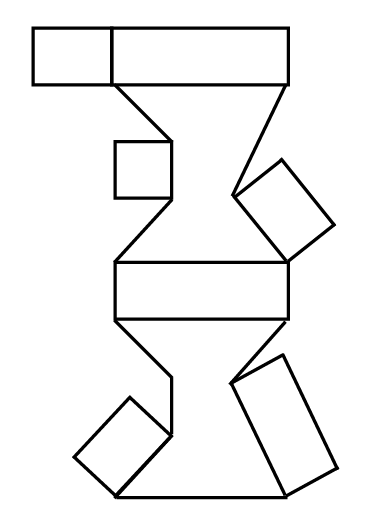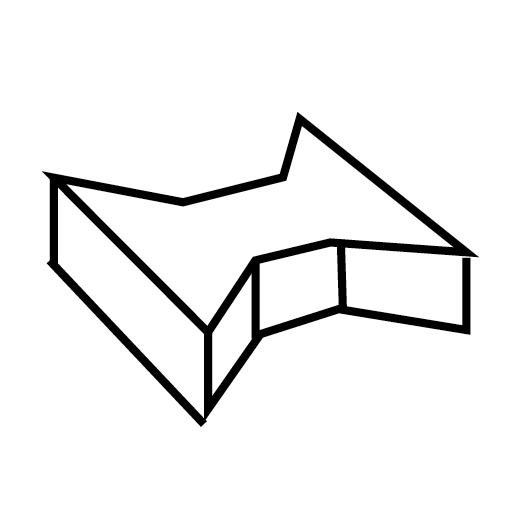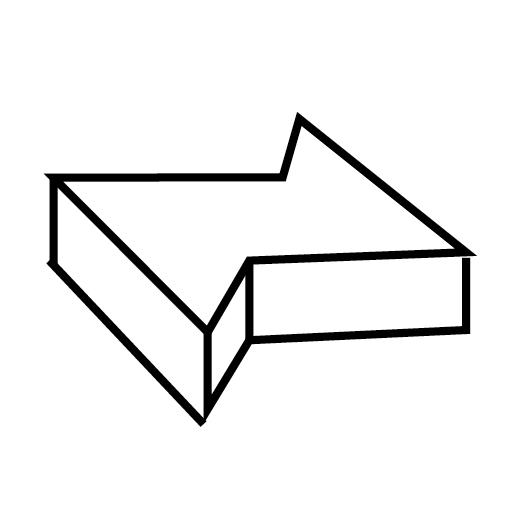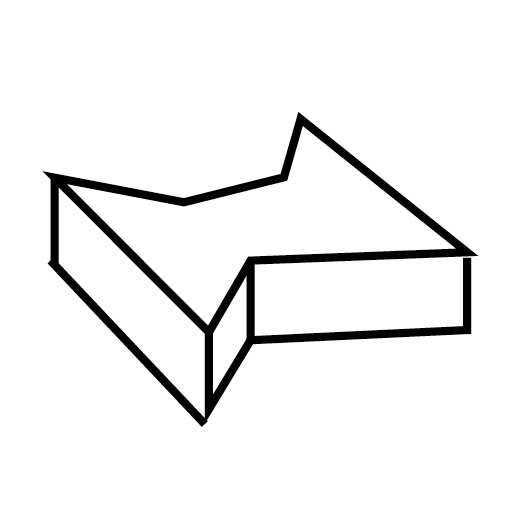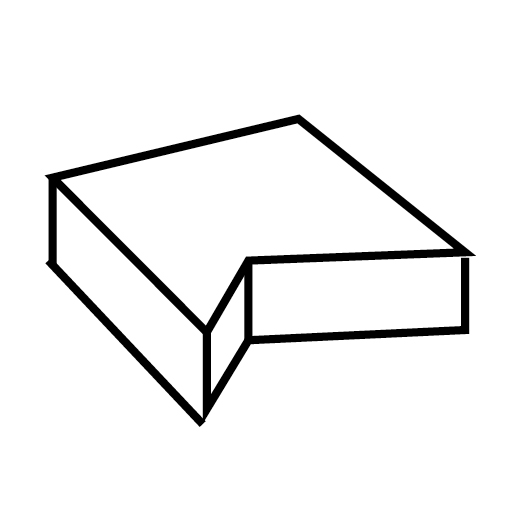Explanation:

To figure out this question, one key detail is particularly important: the largest matching faces of the shape are not symmetrical. On the left side, they have three faces, and on the right side, they have only two. The two longer horizontal rectangles fold up into the height of the shape separating these two larger faces from one another, and the four remaining "flaps" fold down to create the heights of each of the angled faces.

Noticing the detail about the mismatched number of faces is enough information to allow you to solve this problem without picturing how the rest of the shape comes together through folding, as all of the answer choices result in a similar, prism-like shape.This shape has two sides in the side facing the view, but it also has two sides on the opposite side of the shape, so it can't be correct. One of those sides would need to have three faces, not two, for the shape to be correct.This shape is also incorrect. While the side nearest us has two faces, the opposing side only has one. Again, it would need three to be correct.This shape is incorrect as well. The side facing us in this view has three faces, but so does the opposite side. It would need to have two for the shape to be correct.This is the correct shape. The side facing us has two faces, and the side facing away from us has three faces. The faces are the same lengths as shown in the flat diagram.

### Example Question #2 : 3 D Form Development / Pattern Folding Questions

In this question, a flat pattern is presented. This pattern is to be folded into a three-dimensional figure. The correct figure is one of the four given as answer choices. There is only one correct figure in each set. The outside of the folded shape is what is seen as the flat pattern. Select the three-dimensional shape that the flat pattern produces when folded.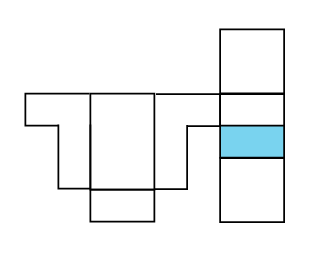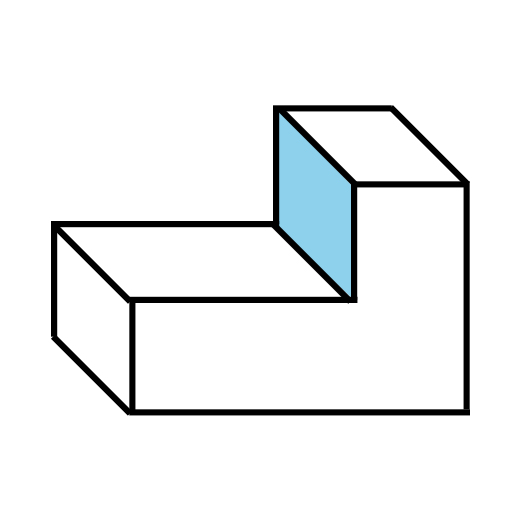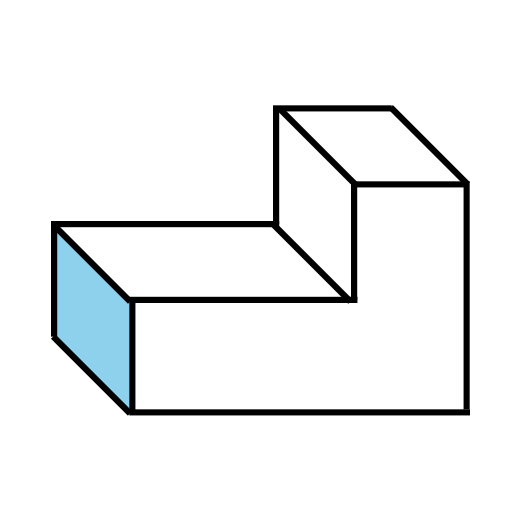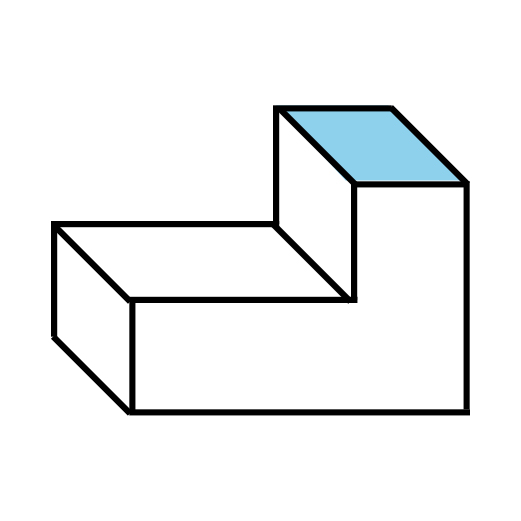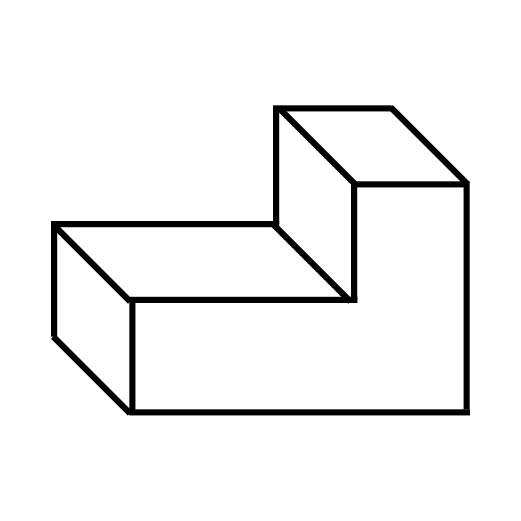Explanation:

In this 3-D Form Development / Pattern Folding question, we need to figure out which face of the resulting L-shaped figure corresponds with the blue face on the flat diagram. We can ignore the answer choice that does not show any visible face as the blue one, because the only faces hidden from the shown view are larger than the rectangular face shown in the diagram. One would be the large square, and the other would be one of the large L-shaped faces.

Looking at the flat diagram, it's easy to see we have three similar rectangular faces to choose from, which correlate with the remaining three answer choices. If the L-shaped large faces are opposite one another, the rectangular shape shown underneath the large rectangle on the left of the flat diagram has to correlate to the one found on the far left of the folded shape. This isn't the blue rectangle, so the following answer choice is incorrect:The rectangle that is directly adjacent to the right L-shaped face in the flat diagram has to share an edge/fold with it. This means that this has to be the top of the L-shape. Since it's not blue either, the following answer choice is also incorrect:The only remaining answer choice is the correct one. The blue rectangle is the one underneath the rectangle adjacent to the L-shape. You can picture how if the white rectangle forms the top of the L-shape, the blue one is folded such that it becomes the height of the vertical section of the "L." This means that the following shape is the correct answer:### All DAT Perceptual Ability Resources